Download PresentationR Tutorial For Beginners | R Programming Tutorial l R Language For Beginners | R Training | Edureka

R Tutorial For Beginners | R Programming Tutorial l R Language For Beginners | R Training | Edureka - PowerPoint PPT Presentation

This Edureka R Tutorial (R Tutorial Blog: https://goo.gl/mia382) will help you in understanding the fundamentals of R tool and help you build a strong foundation in R. Below are the topics covered in this tutorial:

1. Why do we need Analytics ?
2. What is Business Analytics ?
3. Why R ?
4. Variables in R
5. Data Operator
6. Data Types
7. Flow Control
8. Plotting a graph in RI am the owner, or an agent authorized to act on behalf of the owner, of the copyrighted work described.
Presentation TranscriptEDUREKA DATA ANALYTICS WITH R CERTIFICATION TRAINING

https://www.edureka.co/r-for-analytics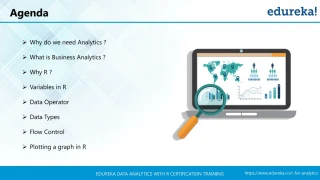Agenda

➢ Why do we need Analytics ?

➢ What is Business Analytics ?

➢ Why R ?

➢ Variables in R

➢ Data Operator

➢ Data Types

➢ Flow Control

➢ Plotting a graph in R

https://www.edureka.co/r-for-analytics

EDUREKA DATA ANALYTICS WITH R CERTIFICATION TRAININGWhy do we need Analytics?

https://www.edureka.co/r-for-analytics

EDUREKA DATA ANALYTICS WITH R CERTIFICATION TRAINING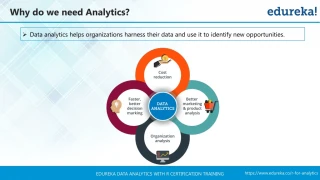Why do we need Analytics?

➢ Data analytics helps organizations harness their data and use it to identify new opportunities.

https://www.edureka.co/r-for-analytics

EDUREKA DATA ANALYTICS WITH R CERTIFICATION TRAINING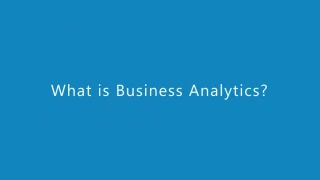https://www.edureka.co/r-for-analytics

EDUREKA DATA ANALYTICS WITH R CERTIFICATION TRAINING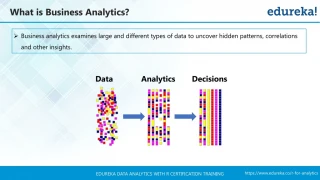➢ Business analytics examines large and different types of data to uncover hidden patterns, correlations

and other insights.

Data

Analytics

Decisions

https://www.edureka.co/r-for-analytics

EDUREKA DATA ANALYTICS WITH R CERTIFICATION TRAINING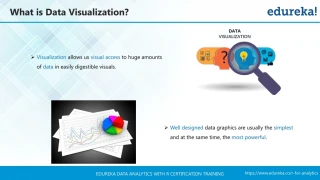What is Data Visualization?

of data in easily digestible visuals.

➢ Well designed data graphics are usually the simplest

and at the same time, the most powerful.

https://www.edureka.co/r-for-analytics

EDUREKA DATA ANALYTICS WITH R CERTIFICATION TRAINING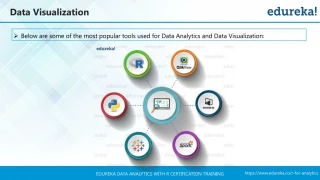Data Visualization

➢ Below are some of the most popular tools used for Data Analytics and Data Visualization:

https://www.edureka.co/r-for-analytics

EDUREKA DATA ANALYTICS WITH R CERTIFICATION TRAININGWhy R?

https://www.edureka.co/r-for-analytics

EDUREKA DATA ANALYTICS WITH R CERTIFICATION TRAINING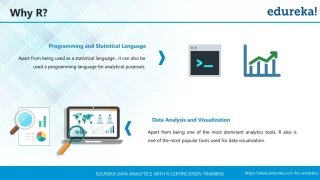Why R?

Programming and Statistical Language

Apart from being used as a statistical language , it can also be

used a programming language for analytical purposes.

Data Analysis and Visualization

Apart from being one of the most dominant analytics tools, R also is

one of the most popular tools used for data visualization.

https://www.edureka.co/r-for-analytics

EDUREKA DATA ANALYTICS WITH R CERTIFICATION TRAININGWhy R?

Simple and Easy to Learn

R is a simple and easy to learn, read & write

Free and Open Source

R is an example of a FLOSS (Free/Libre and Open Source Software)

which means one can freely distribute copies of this software, read it's

source code, modify it, etc.

https://www.edureka.co/r-for-analytics

EDUREKA DATA ANALYTICS WITH R CERTIFICATION TRAININGInstallation Steps

https://www.edureka.co/r-for-analytics

EDUREKA DATA ANALYTICS WITH R CERTIFICATION TRAININGR Installation

Go to https://cran.r-

project.org

1

2

https://www.edureka.co/r-for-analytics

EDUREKA DATA ANALYTICS WITH R CERTIFICATION TRAININGRStudio IDE Installation

Go to

https://www.rstudio.com/

1

C/C++

2

https://www.edureka.co/r-for-analytics

EDUREKA DATA ANALYTICS WITH R CERTIFICATION TRAININGFundamental Concepts of R

Selection

Statements

Data Operator

Data Types

Loops

Variables

https://www.edureka.co/r-for-analytics

EDUREKA DATA ANALYTICS WITH R CERTIFICATION TRAININGVariables in R

➢ Variables are nothing but reserved memory locations to store values. This means that when you

create a variable you reserve some space in memory.

Memory

X = 25

X = 25

Y <-

“Hello”

TRUE -> B

Y = Hellp

B = TRUE

https://www.edureka.co/r-for-analytics

EDUREKA DATA ANALYTICS WITH R CERTIFICATION TRAININGFundamental Concepts of R

Data Operators

Selection

Statements

Variables

Data Types

Loops

https://www.edureka.co/r-for-analytics

EDUREKA DATA ANALYTICS WITH R CERTIFICATION TRAININGData Operators in R

1

Arithmetic Operators

2

Assignment Operators

3

Relational Operators

4

Logical Operators

5

Special Operators

https://www.edureka.co/r-for-analytics

EDUREKA DATA ANALYTICS WITH R CERTIFICATION TRAININGArithmetic Operators

+

>> 2 + 3

5

>> +2

Add two operands or unary plus

1

Arithmetic Operators

-

>> 3 – 1

2

>> -2

Subtract two operands or unary

subtract

2

Assignment Operators

Relational Operators

3

*

>> 2 * 3

6

Multiply two operands

4

Logical Operators

5

Special Operators

/

Divide left operand with the right and result

is in float

>> 6 / 3

2.0

https://www.edureka.co/r-for-analytics

EDUREKA DATA ANALYTICS WITH R CERTIFICATION TRAININGArithmetic Operators

^

1

Arithmetic Operators

>> 2 ^ 3

8

Left operand raised to the power of right

2

Assignment Operators

%%

Remainder of the division of left operand

by the right

>> 5 %% 2

1

Relational Operators

3

4

Logical Operators

%/%

Division that results into whole number

adjusted to the left in the number line

>> 7 %/ %3

2

5

Special Operators

https://www.edureka.co/r-for-analytics

EDUREKA DATA ANALYTICS WITH R CERTIFICATION TRAININGAssignment Operators

=

>> x=5

>>x

5

x = <right operand>

1

Arithmetic Operators

<−

>> x<- 15

>> x

15

2

Assignment Operators

x <- <right operand>

Relational Operators

3

<<−

>> x <<- 2

>> x

2

x <<- <right operand>

Logical Operators

4

5

Special Operators

->

>> 25 -> x

>> x

25

<left operand> -> x

https://www.edureka.co/r-for-analytics

EDUREKA DATA ANALYTICS WITH R CERTIFICATION TRAININGRelational Operators

>

>> 2 > 3

False

True if left operand is greater than the right

1

Arithmetic Operators

<

>> 2 < 3

True

2

Assignment Operators

True if left operand is less than the right

3

Relational Operators

==

>>2 == 2

True

True if left operand is equal to right

Logical Operators

4

5

Special Operators

!=

>> x >>=

2

>>print(x)

1

True if left operand is not equal to the right

https://www.edureka.co/r-for-analytics

EDUREKA DATA ANALYTICS WITH R CERTIFICATION TRAININGRelational Operators

1

Arithmetic Operators

>=

>> 2 > =3

False

True if left operand is greater than or equal

to the right operand

2

Assignment Operators

3

Relational Operators

=<

>> 2 =< 3

True

Logical Operators

4

True if left operand is less than or equal to

the right operand

5

Special Operators

https://www.edureka.co/r-for-analytics

EDUREKA DATA ANALYTICS WITH R CERTIFICATION TRAININGLogical Operators

&

>> 2 & 3

3

1

Arithmetic Operators

Returns x if x is False , y otherwise

2

Assignment Operators

|

>> 2 | 3

2

Returns y if x is False, x otherwise

3

Relational Operators

4

Logical Operators

!

>> ! 1

False

Returns True if x is True, False otherwise

5

Special Operators

https://www.edureka.co/r-for-analytics

EDUREKA DATA ANALYTICS WITH R CERTIFICATION TRAININGSpecial Operators

1

Arithmetic Operators

:

>> x <- 2:8

>> x

2 3 4 5 6 7 8

It creates the series of numbers in sequence

for a vector

2

Assignment Operators

3

Relational Operators

%in%

>> x <- 2:8

>> y <- 5

>>y %in% x

True

4

Logical Operators

This operator is used to identify if an element

belongs to a vector.

5

Special Operators

https://www.edureka.co/r-for-analytics

EDUREKA DATA ANALYTICS WITH R CERTIFICATION TRAININGFundamental Concepts of R

Selection

Statements

Data Operator

Variable

Loops

Data Types

https://www.edureka.co/r-for-analytics

EDUREKA DATA ANALYTICS WITH R CERTIFICATION TRAININGData Type

➢ We do not need to declare a variables before using them.

C/C++

https://www.edureka.co/r-for-analytics

EDUREKA DATA ANALYTICS WITH R CERTIFICATION TRAININGData Type

Vectors

➢ A Vector is a sequence of data elements of the same basic type.

Example:

vtr = (1, 3, 5 ,7 9)

or

vtr <- (1, 3, 5 ,7 9)

Lists

Arrays

Matrices

Factors

➢ There are 5 Atomic vectors, also termed as five classes of vectors.

Data Frames

https://www.edureka.co/r-for-analytics

EDUREKA DATA ANALYTICS WITH R CERTIFICATION TRAININGData Type

Vectors

Lists

Arrays

Matrices

Factors

Data Frames

https://www.edureka.co/r-for-analytics

EDUREKA DATA ANALYTICS WITH R CERTIFICATION TRAININGData Type

Sequence Operations:

Vectors

➢ Indexing

Lists

List(2:4)

list <- c(“a” , “b” , “c” ,”d”)

“b” “c” “d”

Arrays

➢ Replacing

Matrices

“a” , “f” , “c” ,”d”

list <- c(“a” , “b” , “c” ,”d”)

list<- “f’

Factors

➢ sort()

Data Frames

list <- c(4 , 6, 3, 8, 1)

Sorted<- sort(list)

1 3 4 6 8

https://www.edureka.co/r-for-analytics

EDUREKA DATA ANALYTICS WITH R CERTIFICATION TRAININGData Type

Vectors

➢ Lists are the R objects which contain elements of different types like

− numbers, strings, vectors and another list inside it.

Lists

Arrays

> n = c(2, 3, 5)

> s = c("aa", "bb", "cc", "dd", "ee")

>x = list(n, s, TRUE)

Matrices

Factors

Data Frames

https://www.edureka.co/r-for-analytics

EDUREKA DATA ANALYTICS WITH R CERTIFICATION TRAININGData Type

Sequence Operations:

Vectors

➢ Merging

list1 <- list(1,2,3)

list2 <- list("Sun","Mon","Tue")

1 2 3

Lists

merged.list <- c(list1,list2)

Sun Mon Tue

Arrays

➢ Slicing

list1 <- list(1,2,3)

list2 <- list("Sun","Mon","Tue")

List3 <- c(list1, list2)

Matrices

List3

Sun Mon Tue

Factors

➢ Indexing

Data Frames

string1[-1] + string

list1 <- list(1,2,3)

‘da’

https://www.edureka.co/r-for-analytics

EDUREKA DATA ANALYTICS WITH R CERTIFICATION TRAININGData Type

Vectors

➢ Arrays are the R data objects which can store data in more than two dimensions.

➢ It takes vectors as input and uses the values in the dim parameter to create an

array.

Lists

Arrays

Matrices

vector1 <- c(5,9,3)

vector2 <- c(10,11,12,13,14,15)

result <- array(c(vector1,vector2),dim = c(3,3,2))

Factors

Data Frames

https://www.edureka.co/r-for-analytics

EDUREKA DATA ANALYTICS WITH R CERTIFICATION TRAININGData Type

Vectors

➢ Matrices are the R objects in which the elements are arranged in a two-

dimensional rectangular layout.

➢ A Matrix is created using the matrix() function.

Lists

Arrays

matrix(data, nrow, ncol, byrow, dimnames)

Matrices

❖ data is the input vector which becomes the data elements of the matrix.

❖ nrow is the number of rows to be created.

❖ ncol is the number of columns to be created.

❖ byrow is a logical clue. If TRUE then the input vector elements are arranged by row.

❖ dimname is the names assigned to the rows and columns.

Factors

Data Frames

https://www.edureka.co/r-for-analytics

EDUREKA DATA ANALYTICS WITH R CERTIFICATION TRAININGData Type

Vectors

➢ Factors are the data objects which are used to categorize the data and store it as

levels

➢ They can store both strings and integers.

➢ They are useful in data analysis for statistical modeling.

Lists

Arrays

Matrices

Factors

data <- c("East","West","East","North","North","East","West","West“,"East“)

factor_data <- factor(data)

Data Frames

https://www.edureka.co/r-for-analytics

EDUREKA DATA ANALYTICS WITH R CERTIFICATION TRAININGData Type

Vectors

➢ A data frame is a table or a two-dimensional array-like structure in which each

column contains values of one variable and each row contains one set of values

from each column.

Lists

Arrays

emp_id = c (1:5),

Matrices

emp_name = c("Rick","Dan","Michelle","Ryan","Gary"),

salary = c(623.3,515.2,611.0,729.0,843.25),

emp.data <- data.frame(emp_id, emp_name, salary)

Factors

Data Frames

https://www.edureka.co/r-for-analytics

EDUREKA DATA ANALYTICS WITH R CERTIFICATION TRAININGFlow Control Statements

https://www.edureka.co/r-for-analytics

EDUREKA DATA ANALYTICS WITH R CERTIFICATION TRAININGFlow Control Statements

https://www.edureka.co/r-for-analytics

EDUREKA DATA ANALYTICS WITH R CERTIFICATION TRAININGFundamental Concepts of R

Selection

Statements

Data Operator

Variable

Loops

Data Types

https://www.edureka.co/r-for-analytics

EDUREKA DATA ANALYTICS WITH R CERTIFICATION TRAININGFlow Control

SELECTORS CHOOSE ACTIONS

SO YOU DON’T HAVE TO ...

It evaluates a single

condition

if

It evaluates a group of

conditions and selects the

statements

if .. else

It checks the different

known possibilities and

selects the statements

switch

https://www.edureka.co/r-for-analytics

EDUREKA DATA ANALYTICS WITH R CERTIFICATION TRAININGFlow Control

1. If statement

START

Syntax:

if (condition):

statements 1 …

if condition

TRUE

FALSE

Conditional code

End

https://www.edureka.co/r-for-analytics

EDUREKA DATA ANALYTICS WITH R CERTIFICATION TRAININGFlow Control

2. if…else statement

Start

Syntax:

if (condition 1):

statements 1 …

.

.

.

else

statements n …

FALSE

If

Else If

Condition

Condition

FALSE

TRUE

TRUE

Else code

If code

Else If code

End

https://www.edureka.co/r-for-analytics

EDUREKA DATA ANALYTICS WITH R CERTIFICATION TRAININGFlow Control

START

3. Switch statement

Syntax:

Switch

switch (expression,

value1: Statement1

value2: Statement2

.

.

, default Statement

)

TRUE

Case1

Statement1

FALSE

TRUE

Statement2

Case2

FALSE

Default

Statement

default

End

https://www.edureka.co/r-for-analytics

EDUREKA DATA ANALYTICS WITH R CERTIFICATION TRAININGFundamental Concepts of R

Conditional

Statements

Data Operator

Variable

Data Types

Loops

https://www.edureka.co/r-for-analytics

EDUREKA DATA ANALYTICS WITH R CERTIFICATION TRAININGFlow Control

LOOPS REPEAT ACTIONS

SO YOU DON’T HAVE TO ...

Repeat things until the

loop condition is true

Repeat

Repeat things until the

loop condition is true

While

Repeat things till the

given number of times

For

https://www.edureka.co/r-for-analytics

EDUREKA DATA ANALYTICS WITH R CERTIFICATION TRAININGFlow Control

4. repeat statement

START

Syntax:

repeat {

commands

if(condition) {

break

}

}

EXECUTE BLOCK

repeat

TRUE

FALSE

CHECK

CONDITION

EXIT LOOP

https://www.edureka.co/r-for-analytics

EDUREKA DATA ANALYTICS WITH R CERTIFICATION TRAININGFlow Control

5. while statement

START

Syntax:

while (condition is True)

{

statements…

}

FALSE

CHECK

CONDITION

TRUE

repeat

EXIT LOOP

EXECUTE BLOCK

https://www.edureka.co/r-for-analytics

EDUREKA DATA ANALYTICS WITH R CERTIFICATION TRAININGFlow Control

6. for statement

START

Syntax:

for(value in vector)

{

statements…

}

Initialization

FALSE

Check

condition

Exit loop

TRUE

repeat

Execute Statements

https://www.edureka.co/r-for-analytics

EDUREKA DATA ANALYTICS WITH R CERTIFICATION TRAININGFlow Control

7. break

START

Syntax:

break;

FALSE

Check Loop

Condition

TRUE

EXIT LOOP

repeat

TRUE

Check Break

Condition

FALSE

EXIT LOOP

Execute Block

https://www.edureka.co/r-for-analytics

EDUREKA DATA ANALYTICS WITH R CERTIFICATION TRAININGFlow Control

8. next

START

Syntax:

next;

FALSE

Check Loop

Condition

TRUE

EXIT LOOP

repeat

Execute Block 1

Check next

Condition

TRUE

FALSE

Execute Block 2

https://www.edureka.co/r-for-analytics

EDUREKA DATA ANALYTICS WITH R CERTIFICATION TRAININGData Visualization in R

https://www.edureka.co/r-for-analytics

EDUREKA DATA ANALYTICS WITH R CERTIFICATION TRAININGData Visualization in R

➢ Data Visualization helps the organizations unleash the power of their most valuable assets: their

data and their people.

Data Visualization

Pie Chart

Bar Chart

Boxplot

Histogram

Line Graph

Scatterplot

https://www.edureka.co/r-for-analytics

EDUREKA DATA ANALYTICS WITH R CERTIFICATION TRAININGData Visualization

➢ Pie charts are best to use when you are trying to compare parts of a

whole.

Pie Chart

Bar Chart

Boxplot

Histogram

Line Graph

Scatterplot

https://www.edureka.co/r-for-analytics

EDUREKA DATA ANALYTICS WITH R CERTIFICATION TRAININGData Visualization

➢ Bar graphs are used to compare things between different groups or

to track changes over time.

Pie Chart

Bar Chart

Boxplot

Histogram

Line Graph

Scatterplot

https://www.edureka.co/r-for-analytics

EDUREKA DATA ANALYTICS WITH R CERTIFICATION TRAININGData Visualization

➢ Boxplot are used summarize data from multiple sources and display

the results in a single graph.

Pie Chart

Bar Chart

Boxplot

Histogram

Line Graph

Scatterplot

https://www.edureka.co/r-for-analytics

EDUREKA DATA ANALYTICS WITH R CERTIFICATION TRAININGData Visualization

➢ Histogram are used to plot the frequency of score occurrences in a

continuous data set that has been divided into classes, called bins.

Pie Chart

Bar Chart

Boxplot

Histogram

Line Graph

Scatterplot

https://www.edureka.co/r-for-analytics

EDUREKA DATA ANALYTICS WITH R CERTIFICATION TRAININGData Visualization

➢ Line graphs are used to track changes over short and long periods

of time.

Pie Chart

Bar Chart

Boxplot

Histogram

Line Graph

Scatterplot

https://www.edureka.co/r-for-analytics

EDUREKA DATA ANALYTICS WITH R CERTIFICATION TRAININGData Visualization

➢ Scatter plots show how much one variable is affected by another.

Pie Chart

Bar Chart

Boxplot

Histogram

Line Graph

Scatterplot

https://www.edureka.co/r-for-analytics

EDUREKA DATA ANALYTICS WITH R CERTIFICATION TRAININGSummary

https://www.edureka.co/r-for-analytics

EDUREKA DATA ANALYTICS WITH R CERTIFICATION TRAININGSession In A Minute

What is Data Analysis?

Why do we need Analytics?

Why R?

Data Operators in R

Data Types in R

Flow Control Statement

https://www.edureka.co/r-for-analytics

EDUREKA DATA ANALYTICS WITH R CERTIFICATION TRAININGThat’s all folks!

That’s all folks!

https://www.edureka.co/r-for-analytics

EDUREKA DATA ANALYTICS WITH R CERTIFICATION TRAINING